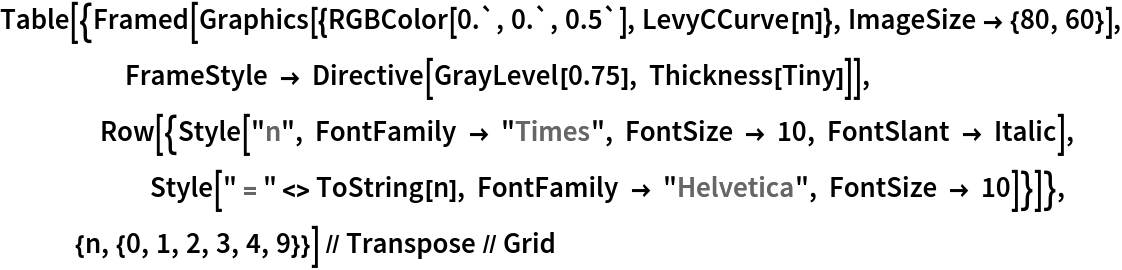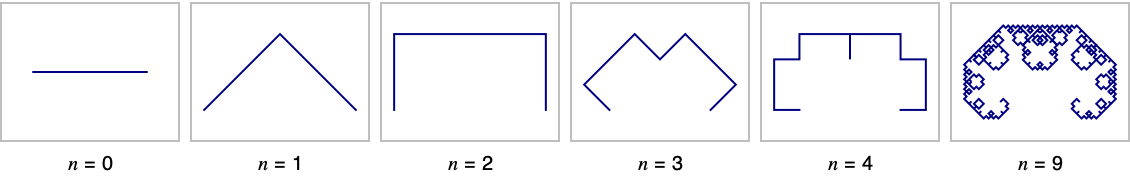Function Repository Resource:

# LevyCCurve

Generate the Lévy C curve

Contributed by: Sander Huisman
 ResourceFunction["LevyCCurve"][n] generates the line segments of the Lévy C curve with n iterations. ResourceFunction["LevyCCurve"][{p1,p2},n] uses the line connecting the points p1 and p2 as the initial line. ResourceFunction["LevyCCurve"][{p1,p2,… ,pn},n] uses the lines joining the points pi.

## Details

The following transformation is done with each line segment: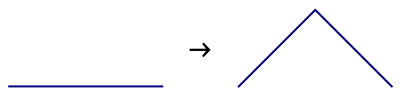The first four iterations and the ninth iteration: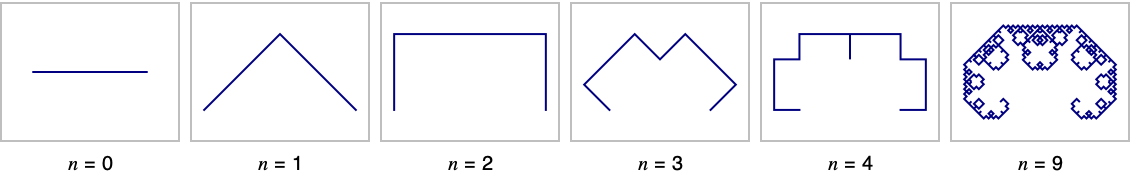ResourceFunction["LevyCCurve"][n] is equivalent to ResourceFunction["LevyCCurve"][{{-1,0},{1,0}},n].
The syntax ResourceFunction["LevyCCurve"][Line[{p1,p2, ,pn}],n] is also supported.

## Examples

### Basic Examples (2)

Generate the Lévy C curve for four iterations:

 In:=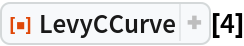Out=Generate the line for eight iterations and visualize the result:

 In:=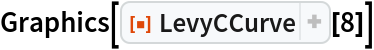Out=### Scope (1)

Start from a vertical line segment:

 In:=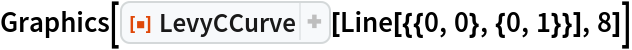Out=### Properties and Relations (1)

The length of the Lévy C curve grows approximately exponentially:

 In:=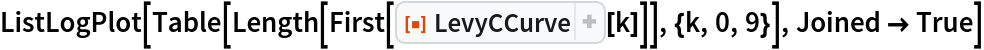Out=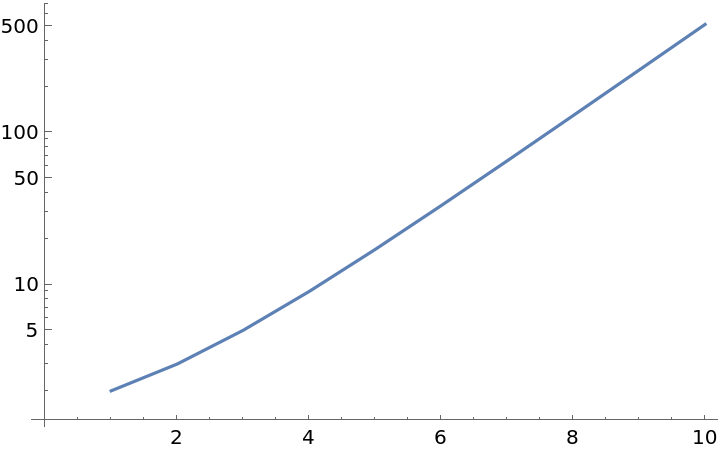### Neat Examples (2)

"Inward" and "outward" Lévy C curve starting from a square:

 In:=Out=Color the curve along its length:

 In:=Out=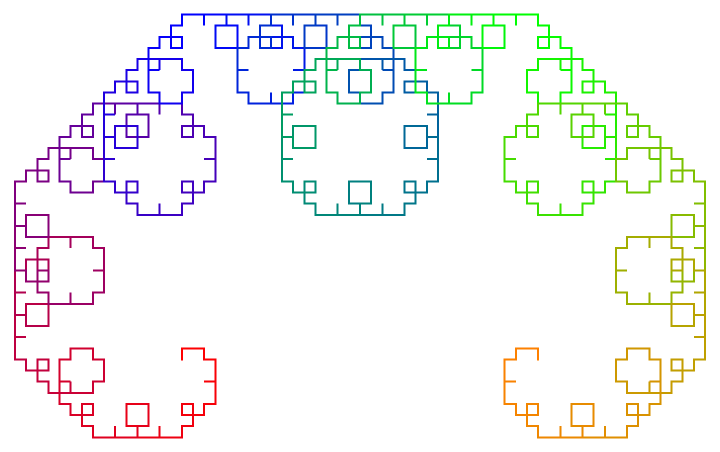SHuisman

## Version History

• 1.0.0 – 22 October 2021

## Author Notes

Code used for images in the Details and Option section:

 In:=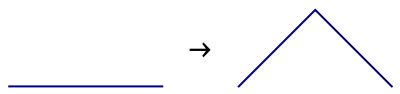In:=